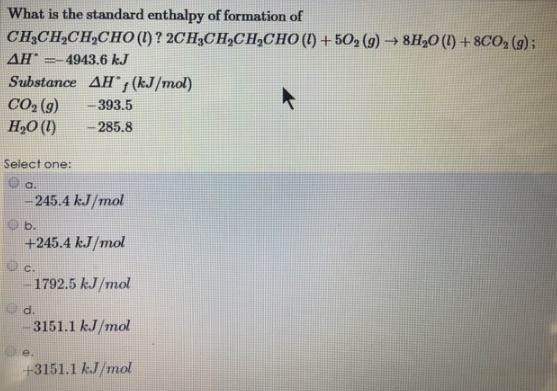# Problem: What is the standard enthalpy of formation of CH3CH2CH2CHO(l)? 2CH3CH2CH2CHO (l) + 5O2 (g) → 8H2O (l) + 8CO2 (g):         ΔH° = -4943.6 kJ Substance             ΔH°f (kJ/mol) CO2 (g)                    - 393.5 H2O (l)                     - 285.8 Select one: a. -245.4 kJ/mol b. +245.4 kJ/mol c. -1792.5 kJ/mol d -3151.1 kJ/mol e. +3151.1 kJ/mol

###### FREE Expert Solution
96% (189 ratings)###### Problem Details

What is the standard enthalpy of formation of CH3CH2CH2CHO(l)?

2CH3CH2CH2CHO (l) + 5O2 (g) → 8H2O (l) + 8CO(g):         ΔH° = -4943.6 kJ

Substance             ΔH°f (kJ/mol)

CO2 (g)                    - 393.5

H2O (l)                     - 285.8

Select one:

a. -245.4 kJ/mol

b. +245.4 kJ/mol

c. -1792.5 kJ/mol

d -3151.1 kJ/mol

e. +3151.1 kJ/mol